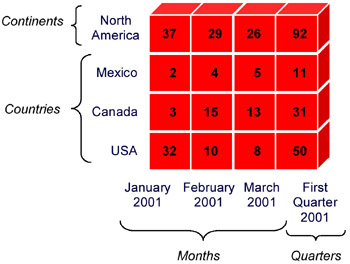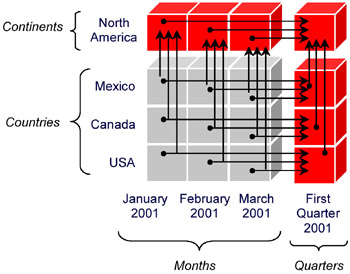# BACKGROUND

Research in multidimensional databases has been extensive (Mendelzon, 2001). In this section we first give a brief overview of a multidimensional database and introduce terminology used in the remainder of the chapter. Next, we outline the treatment of incomplete information in other database models, e.g., the relational model. Finally we consider related work in multidimensional databases.

### Overview of a Multidimensional Database

A useful way of understanding a multidimensional database is to conceive of it as a hierarchy in a multidimensional space. Each dimension is composed of a set of related categories. A category can be thought of as a system of measurement. For example, a Time dimension has categories corresponding to various ways to measure time, such as Months and Quarters, while a Geography dimension could have categories for Countries and Continents. A category is a set of individual measurements called category units, which we will shorten in this chapter to units. In a Time dimension, the units in the Month category would be values such as "January 2001" and "March 2001." In this chapter we use strings to label individual units.

A node in the hierarchy is a point in multidimensional space. The coordinates of a node are a unit chosen from each dimension. Each node contains one or more values, which are called measures. A measure is a quantity being measured. Each measure in a node is the result of an aggregate computed on a set of facts, usually obtained from a database relation. The possible aggregates typically include count, max, min, and sum. To compute a measure in a node, the facts are grouped by unit and the aggregate is applied to the group.

Figure 1 shows an example multidimensional space with two dimensions: Geography and Time. The Time dimension has the categories Months and Quarters. The figure depicts only the units for the first three months in 2001. The Geography dimension has categories for Countries and Continents. The value or measure depicted at each point in the multidimensional space is a count of the number of apples sold at a store in that geographic location during that time.Figure 1: A Multidimensional Space

The hierarchy also has edges. An edge represents a data dependency: the data at the "to" node is derived from the data at the "from" node. An edge connects a unit to a less precise version of itself in another category. For example the hierarchy would have an edge from the unit "January 2001" in the category of Months to the unit "First Quarter 2001" in the category of Quarters. Figure 2 shows the hierarchy for the multidimensional space of Figure 1. The hierarchy has several base nodes with no incoming edges. The measures in the base are computed directly from the underlying data. The base nodes are shaded light gray in Figure 2. The non-base nodes are called derived nodes. The measures in each derived node are the result of an aggregate applied to the measures at nodes on incoming edges. In the example of apple count data, the sum aggregate is used is to add the counts.Figure 2: A Hierarchy in the Multidimensional Space

For the derived nodes to be computable from other nodes, the relationship between a pair of categories must be strict, covering, and onto (Pedersen et al., 2001). Consider the relationship between a fine category and a coarse category. By strict, we mean that the relationship is many-to-one, i.e., a unit in the fine category maps to at most one unit in the coarse category. By covering, we mean that there is an edge from every unit in the fine category to some unit in the coarse category. Said differently, the fine category totally participates in the relationship. Finally, by onto we mean that every unit in the coarse category has some incoming edge from a unit in the fine category. Said differently, the coarse category participates totally in the relationship. The categories, units, and the relationships among them are the metadata in a multidimensional database. Although the cube is a multidimensional space, each dimension is specified in isolation.

A multidimensional database can be implemented using a lazy, eager, or semi-eager strategy (Widom, 1995). The eager strategy materializes every aggregate value in the hierarchy. The advantage of the eager strategy is that values can be quickly fetched from the cube during a query. The primary disadvantage is high storage cost (Shukla et al., 1996, present algorithms for estimating cube size). For many applications, eager cubes are just too big, often 200-500 times the size of the base data (OLAP Report, 2001). A lazy implementation strategy does not materialize values. Instead, the values in the hierarchy are computed from the underlying base data during a query. The disadvantage of the lazy strategy is slower query evaluation (faster evaluation algorithms are being researched, e.g., Agrawal et al., 1996). The semi-eager strategy eagerly materializes only some regions in the multidimensional database, and lazily computes others when needed during a query (Harinarayan et al., 1996), providing a good trade-off between storage use and query response time.Multidimensional Databases: Problems and Solutions
ISBN: 1591400538
EAN: 2147483647
Year: 2003
Pages: 150